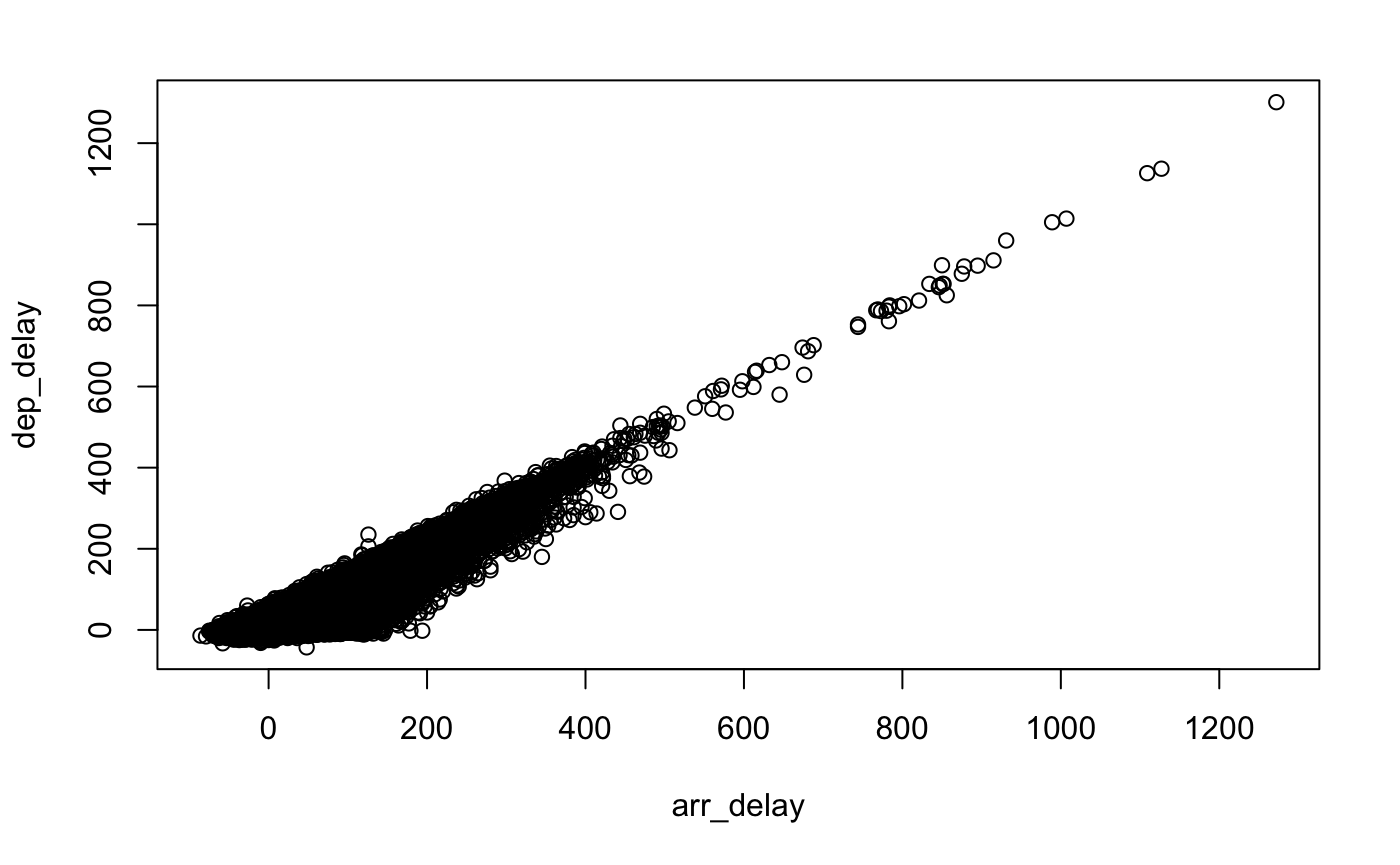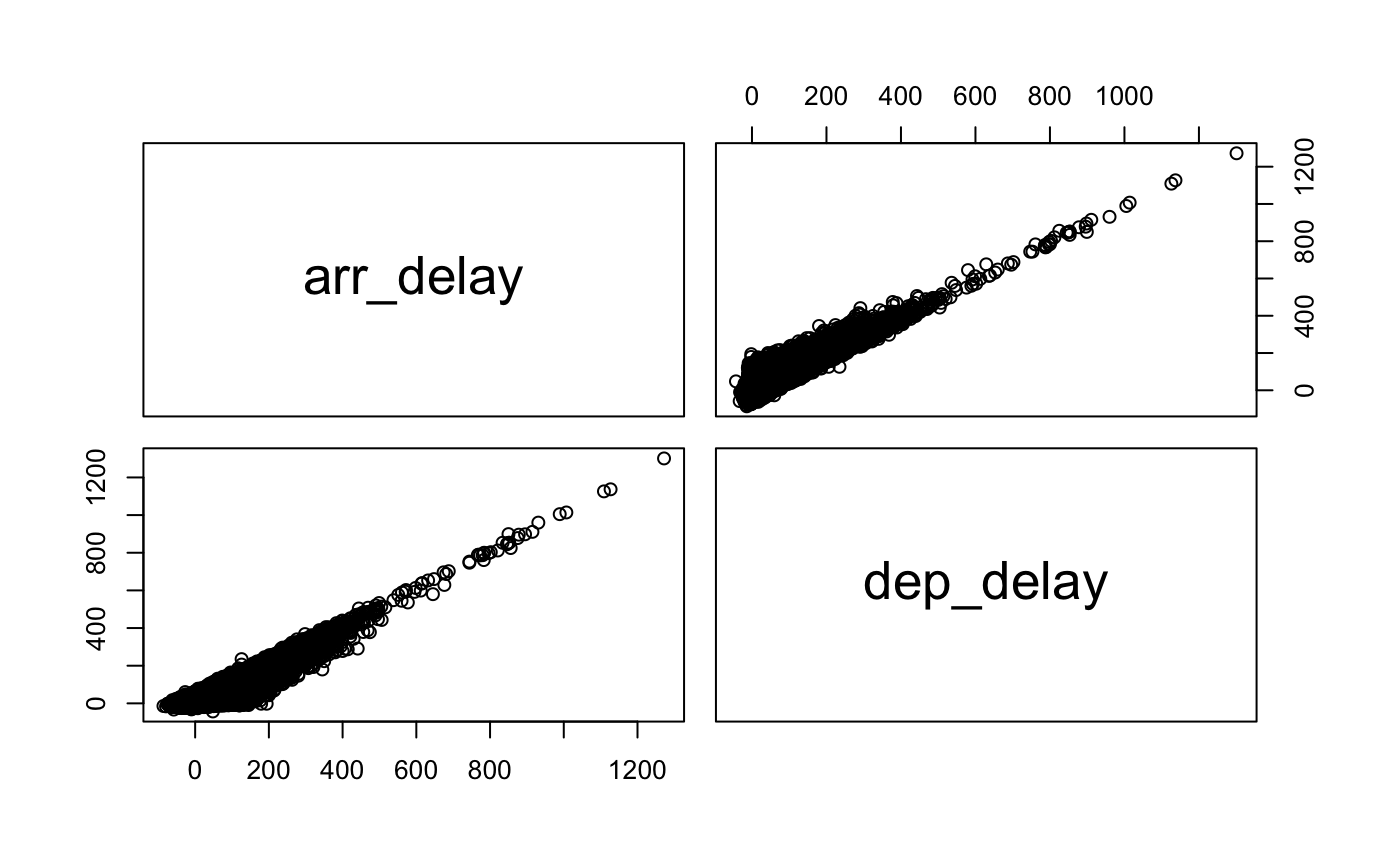# Descriptive Statistics in R

The following notes cover the use of R to create measurements of central tendency: mean(), median() and mode(), as well as the spread of data through range, IQR (inter-quantile-range) and standard deviation.

Finishing the notes is some useful visualizations for this work, including standard R graphic functions, as well as ggplot graphs.

Descriptive Statistics are statistics that explain and explore a dataset. This includes exploring measurements of central tendancy (mean, median, mode) and the spread of the data (standard deviation, variance, etc).

# Central Tednancy

Mean, Median and Mode are the most common measurements of central tendancy. They show where the data congregates as the central aspect of the data.

Mean is the average of data. Median is the middle point of a data set, and in the case of even numbered observations, the two middle values are averaged to create the median. Mode is the most common repeating data value.

In R, these are all built in functions called by their name: mean(), median() and mode()

Data is passed into the functions as a parameter.

# Data Variation

The spread of data is measured in variation and standard deviation. This shows how the data spreads around the center point.

Measurements of variation (or spread) would be: IQR (inter-quantile-range) Range Standard Deviation

Range is simply the difference between the minimum value of a dataset and it’s maximum.

``````library(nycflights13)
flight.data <- nycflights13::flights

range(flight.data\$sched_dep_time)``````
``  106 2359``

IQR is the data range divided up into 4 groups. A simple range will include outliers, where data points (although rare) are extreme and will skew results. IQR helps resolve this by removing the top and low ends of the data range. The inner 2 quarters of IQR will account for 50% of the data. Therefore we know where 50% of the data fits.

``quantile(flight.data\$sched_dep_time)``
``````  0%  25%  50%  75% 100%
106  906 1359 1729 2359 ``````
``IQR(flight.data\$sched_dep_time)``
`` 823``

Notice that while quantile() function derrives all quantile values (0, 25, 75 and 100%), the IQR() function returns the value that is the range in the 50% of the data.

Standard Deviation is a measure of spread around the central tendancy (mean). A small value of of SD means that the data is tight to the central data. A larger value indicates a wider spread of data

``sd(flight.data\$sched_dep_time)``
`` 467.3358``

Keep in mind that in R, the sd() function is only calculating the sample standar deviation. To calculate the population standard deviation, one would need to write their own method.

# Psych Package

The psych package allows for some descriptive statistucs using describe() function as well as the describeBy() function.

Describe is a function that is similar to the standard summary() function but it gives a bit more details and formatting is different. The describeBy() function allows for a built in grouping of data (similar to group_by): describeBy(mydataset, group=variable).

# Visualizing Data

Data visualization is often done with external libraries (such as ggplot2). Yet there are other options.

## Built In Plotting

Within the R language there are standard plotting functions.

The plot() function is an example of a standard visualizaiton that plots a scatter plot:

``plot(arr_delay, dep_delay)``Above we have a simple scatter plot using the plot() function. The first value in the parameters is the X value and the second is the Y.

### Multiple Plots

Multiple plots can be created in R, using the pairs() FORMULA. I called it a formula, because it’s a bit different than a function. Where functions define a calculation to output, a FORMULA defines the relationships you want to investigate.

In R, a formula is defined with the ~. What is on the left of the tilda (if there is something) is the response. On the right of the ~ will be the variable(s) relationships we’re looking at. These variables are separated by a + sign. The + here does not refer to arithmetic. Instead it denotes that these variables will be investigated:

``pairs(~ arr_delay + dep_delay)``Above we have the top right graph which has arr_delay as the Y axis and dep_delay as the X. The bottom left graph has arr_delay as the X axis and dep_delay as the Y axis.

``pairs(~ arr_delay + dep_delay + distance + air_time)``